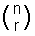## The Binomial Series

This section looks at Binomial Theorem and Pascals Triangle.

Pascal’s Triangle

You should know that (a + b)² = a² + 2ab + b² and you should be able to work out that (a + b)³ = a³ + 3a²b + 3b²a + b³ .
It should also be obvious to you that (a + b)¹ = a + b .

so (a + b)¹  =        a + b
(a + b)² =       a² + 2ab + b²
(a + b)³ =  a³ + 3a²b + 3b²a + b³

You should notice that the coefficients of (the numbers before) a and b are:
1   1
1   2   1
1   3   3   1

If you continued expanding the brackets for higher powers, you would find that the sequence continues:
1   4   6   4   1
1   5  10  10  5   1
1   6  15  20  15  6   1
etc

This sequence is known as Pascal's triangle. Each of the numbers is found by adding together the two numbers directly above it.

So the 20 in the last line is found by adding together 10 and 10. Each of the 10s in the line above are found by adding together a 6 and a 4.

So it is possible to expand (a + b) to any whole number power by knowing Pascal"s triangle.

Example

Find (3 + x)3

The power that we are expanding the bracket to is 3, so we look at the third line of Pascal’s triangle, which is 1 3 3 1.

So the answer is: 33 + 3 × (32 × x) + 3 × (x2 × 3) + x3(we are replacing a by 3 and b by x in the expansion of (a + b)3 above)

Generally

It is, of course, often impractical to write out Pascal"s triangle every time, when all that we need to know are the entries on the nth line. Clearly, the first number on the nth line is 1. The second number is n. The third number is:
n(n - 1)   .
1 × 2

In general, the rth number in the nth line is:
n!        (which is nCr on your calculator)
r! (n - r)!

where n! means ‘n factorial’ and is equal to n × (n-1) × … × 2 × 1

nCr is also often written asand is pronounced “n choose r”.

The Binomial Theorem

The Binomial Theorem states that, where n is a positive integer:

• (a + b)n = an + (nC1)an-1b + (nC2)an-2b2 + … + (nCn-1)abn-1 + bn

Example

Expand (4 + 2x)6 in ascending powers of x up to the term in x3

This means use the Binomial theorem to expand the terms in the brackets, but only go as high as x3.

So to find the answer we substitute 4 for a in the Binomial theorem and 2x for b:

46 + (6C1)(45)(2x) + (6C2)(44)(2x)2 + (6C3)(43)(2x)3 + …
= 4096 + (6 ×1024 ×2x) + (15 ×256 ×4x2) + (20 ×64 ×8x3) + …
= 4096 + 12288x + 15360x2 + 10240x3 + …

The Binomial Theorem for (1 + x)n

The previous version of the binomial theorem only works when n is a positive integer. If n is any fraction, the binomial theorem becomes:

 (1 + x)n = 1 + nx + n(n - 1)x2 + n(n - 1)(n - 2)x3 + ... 1! 2! 3!

PROVIDING |x| < 1

Note that while the previous series stops, this one goes on forever.

Example

Find the expansion of (5x + 2)1/2

We need to transform this so it looks like (1 + x)1/2, so lets take out a factor of 2:

(5x + 2)1/2 = (2[5x/2 + 1])1/2

Now, where we have 'x' in the above formula, we need 5x/2 and where we have n, we need ½ .

= Ö2(1 + 5x/2)1/2
= Ö2[ 1 + ½ (5x/2) + ½ × ½ (- ½ )(25x2/4) + ... ]

Remember, this is only valid if -1 < 5x/2 < 1, in other words, -2/5 < x < 2/5

Using Partial Fractions

We can expand more complicated expressions, now, using the method of partial fractions where appropriate.

Example

 Expand (6x + 3) (1 + x)(5x + 2)

We can split this up, using partial fractions, into:

1      +     1            .
1 + x       5x + 2

Now expand (1 + x)-1 and (5x + 2)-1 as described above and add.

This video shows how Binomial Distribution works